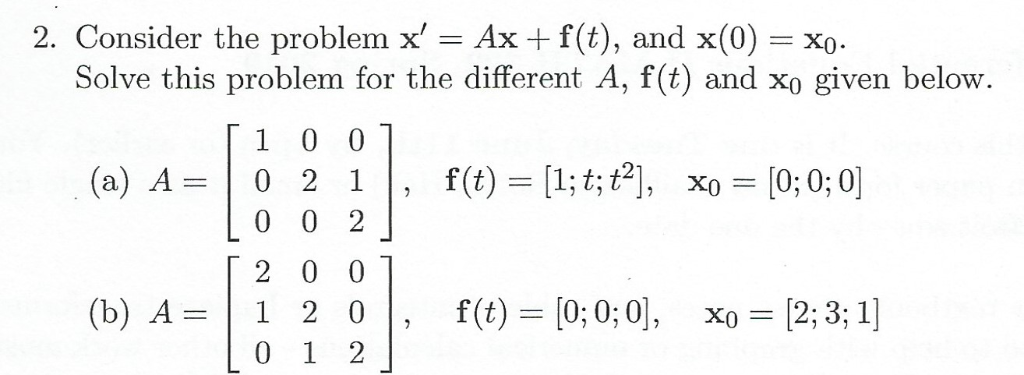# Solved: 2. Consider The Problem X' = Ax F(t), And X(0) Solve This Problem For The Different A, F(t) And Xo Given Below. 1 0 0 F(t) [1; T; T [0; 0; 0 (a) A 0 2 1 0 0 2 2 0 0 F(t) [0; 0; 0 (b) A = 0x =

By |2. Consider the problem x' = Ax f(t), and x(0) Solve this problem for the different A, f(t) and xo given below. 1 0 0 f(t) [1; t; t [0; 0; 0 (a) A 0 2 1 0 0 2 2 0 0 f(t) [0; 0; 0 (b) A = 0x = [2; 3; 1] 1 20 - O 1 2